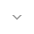belongs to Maven artifact com.android.support:support-compat:28.0.0-alpha1

# MathUtils

``` public class MathUtils ```
``` extends Object ``` ``` ```

 java.lang.Object ↳ android.support.v4.math.MathUtils

A utility class providing functions useful for common mathematical operations.

## Summary

### Public methods

``` static double``` ``` clamp(double value, double min, double max) ```

This method takes a numerical value and ensures it fits in a given numerical range.

``` static float``` ``` clamp(float value, float min, float max) ```

This method takes a numerical value and ensures it fits in a given numerical range.

``` static int``` ``` clamp(int value, int min, int max) ```

This method takes a numerical value and ensures it fits in a given numerical range.

### Inherited methodsFrom class ``` java.lang.Object ```

## Public methods

### clamp

```double clamp (double value,
double min,
double max)```

This method takes a numerical value and ensures it fits in a given numerical range. If the number is smaller than the minimum required by the range, then the minimum of the range will be returned. If the number is higher than the maximum allowed by the range then the maximum of the range will be returned.

Parameters
`value` `double`: the value to be clamped.

`min` `double`: minimum resulting value.

`max` `double`: maximum resulting value.

Returns
`double` the clamped value.

### clamp

```float clamp (float value,
float min,
float max)```

This method takes a numerical value and ensures it fits in a given numerical range. If the number is smaller than the minimum required by the range, then the minimum of the range will be returned. If the number is higher than the maximum allowed by the range then the maximum of the range will be returned.

Parameters
`value` `float`: the value to be clamped.

`min` `float`: minimum resulting value.

`max` `float`: maximum resulting value.

Returns
`float` the clamped value.

### clamp

```int clamp (int value,
int min,
int max)```

This method takes a numerical value and ensures it fits in a given numerical range. If the number is smaller than the minimum required by the range, then the minimum of the range will be returned. If the number is higher than the maximum allowed by the range then the maximum of the range will be returned.

Parameters
`value` `int`: the value to be clamped.

`min` `int`: minimum resulting value.

`max` `int`: maximum resulting value.

Returns
`int` the clamped value.

• ## Classes

[{ "type": "thumb-down", "id": "missingTheInformationINeed", "label":"Missing the information I need" },{ "type": "thumb-down", "id": "tooComplicatedTooManySteps", "label":"Too complicated / too many steps" },{ "type": "thumb-down", "id": "outOfDate", "label":"Out of date" },{ "type": "thumb-down", "id": "samplesCodeIssue", "label":"Samples / code issue" },{ "type": "thumb-down", "id": "otherDown", "label":"Other" }]
[{ "type": "thumb-up", "id": "easyToUnderstand", "label":"Easy to understand" },{ "type": "thumb-up", "id": "solvedMyProblem", "label":"Solved my problem" },{ "type": "thumb-up", "id": "otherUp", "label":"Other" }]